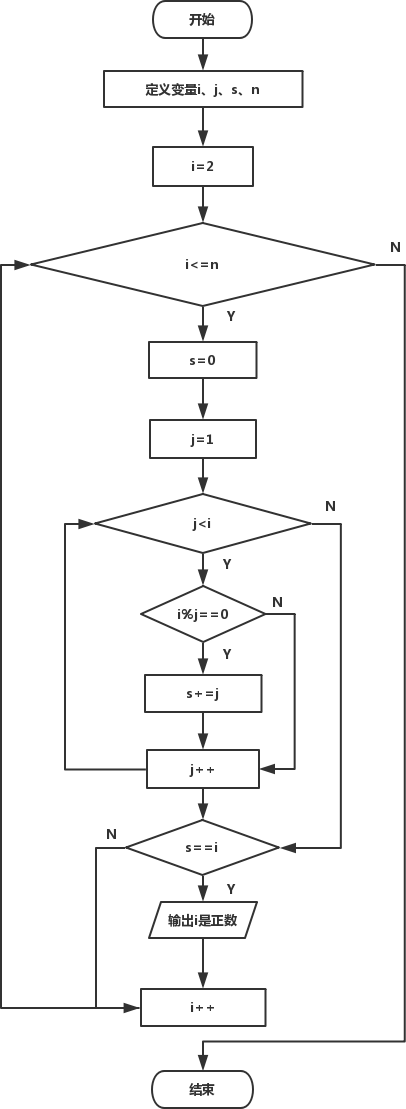• 内容
• 评论
• 相关

## 算法设计```#include<stdio.h>
int main()
{
int i, j, s, n;  /*变量i控制选定数范围，j控制除数范围，s记录累加因子之和*/
printf("请输入所选范围上限：");
scanf("%d", &n);  /* n的值由键盘输入*/
for( i=2; i<=n; i++ )
{
s=0;  /*保证每次循环时s的初值为0*/
for( j=1; j<i; j++ )
{
if(i%j == 0)  /*判断j是否为i的因子*/
s += j;
}
if(s == i)  /*判断因子这和是否和原数相等*/
printf("It's a perfect number:%d\n", i);
}
return 0;
}```

It's a perfect number:6
It's a perfect number:28
It's a perfect number:496
It's a perfect number:8128

## 知识点补充

```#include<stdio.h>>
int main()
{
//...
for( i=2; i<=1000; i++)
{
s=0;
for( j=1; j<=n/2; j++ )
{
if(i%j == 0)
s += j;
}
//...
}
}```

0条评论